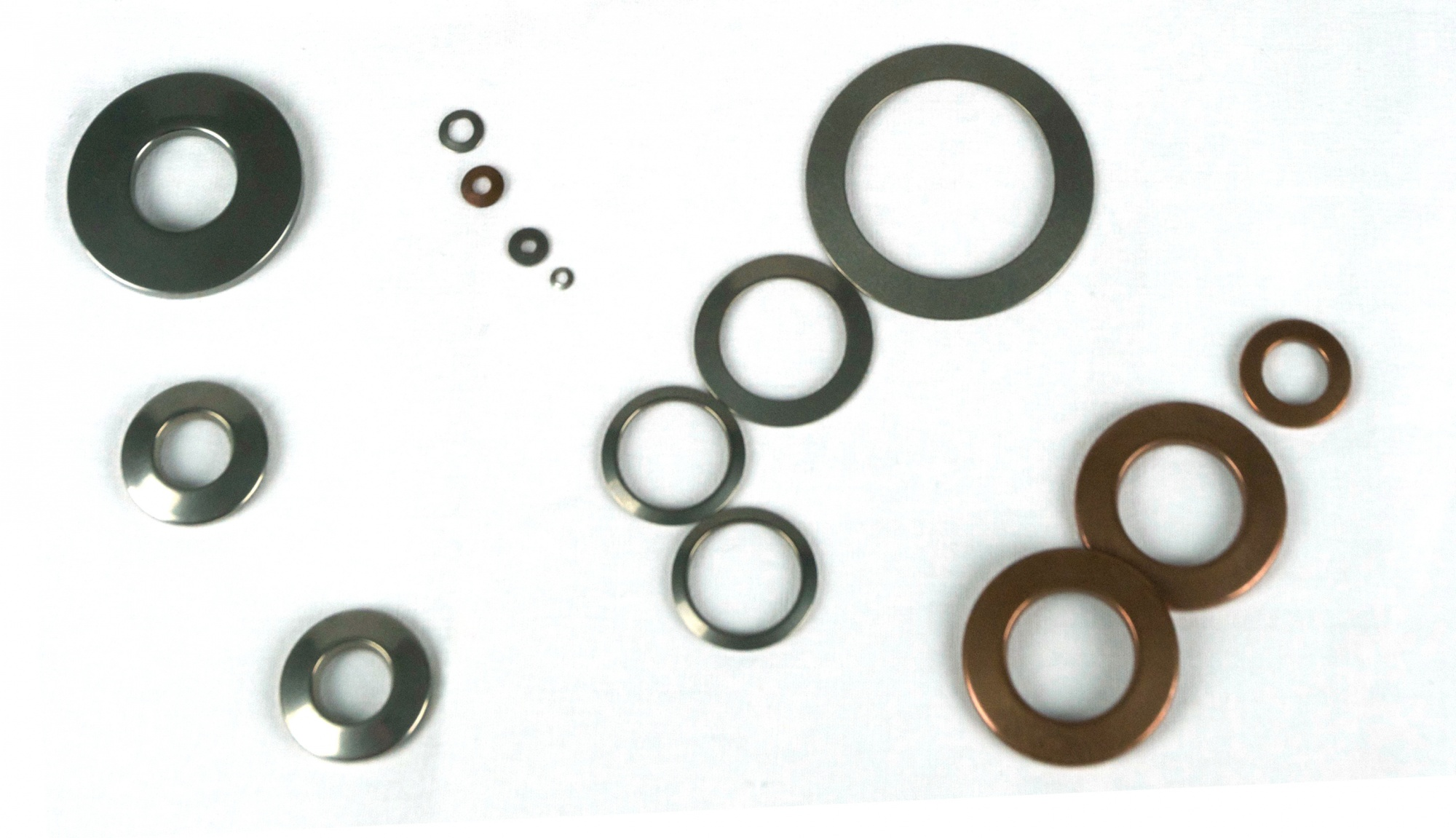## UNITS OF MEASURE

IMPERIAL                                                                   METRIC
Dimensions = inches                                             Dimensions = millimeters
Load = pounds force                                              Load = Newton
Torque = foot-pounds                                          Torque = Newton-meters
Temperature = °F                                                    Temperature = °C

## TORQUE CALCULATION

The widely accepted formula is as follows,

T = K*F*D

where,

K = Estimated nut friction factor
T = Bolt torque
D = Nominal bolt diameter

Solon Manufacturing Co. is migrating to ISO Standard 19690-2 in an effort to standardize our catalog Belleville spring and washer toleraces.